# 一、我的程序效果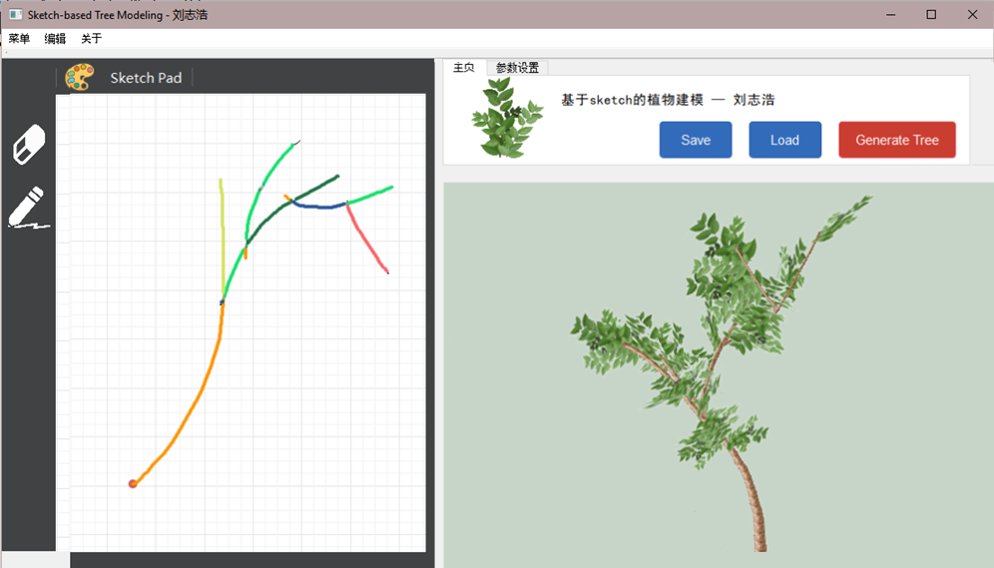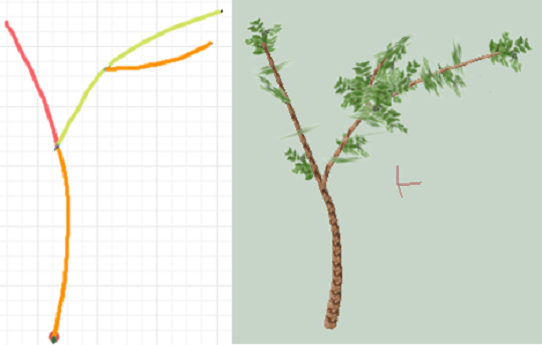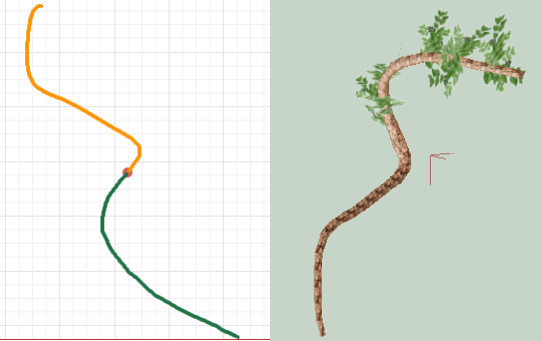# 二、算法流程

## 1 输入绘制的草图## 2 算法综述和数据结构定义

• Branch（枝干）：是树中的一段枝干，它没有任何的分支。在 图 5 中，每条彩色的线段就是一个branch。
• Node（结点）：每条branch由一组node组成，其中P1是该branch与其父枝条的连接点。
• Unit（枝元）：branch的两个相邻结点连接起来，组成一个枝元。这个概念在算法阶段还用不到，主要是为了方便绘制。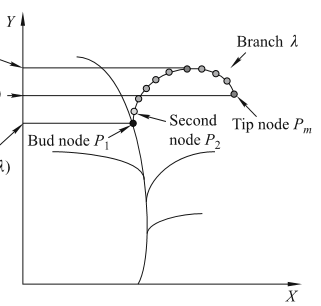## 3. 从草图中划分branch，得到二维骨架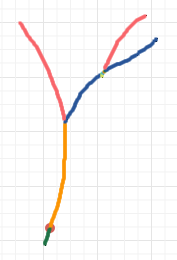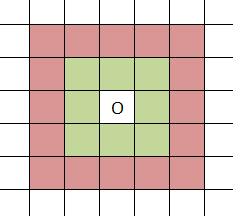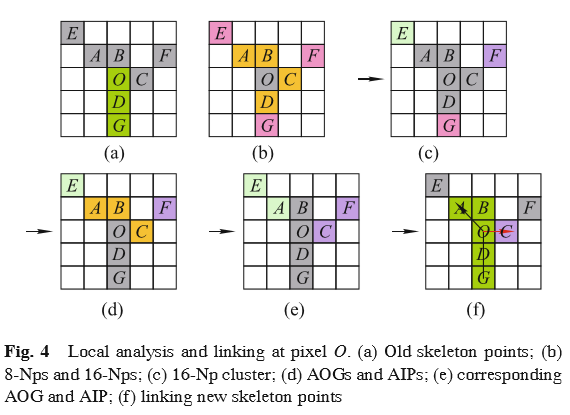1. 如果N=1，把p1直接连接到当前的branch上，并且下一步以p1中心继续进行上面的局部像素分析。
2. 如果N＞1，把p1直接连接到当前的branch上，并且下一步仍然以p1为中心，继续进行上面的局部像素分析。对于其他的新骨架点（pi,i=2,3,…N），都将产生一个新的branch，且pi分别每个新branch的第二个点，它们的第一个点当然是像素O了。如上图的图(f)，A被直接连接到当前的branch上，C则作为新branch的第二个点。
3. 如果N=0，当前branch的搜索结束，换其他还没有搜索过的branch继续进行局部像素分析。

# 三、核心算法

class Branch
{
public:
List<Point> nodes;
List<Branch*> offspring;
} trunk;

void Sketch::generate2DSkeleton(Image image)
{
// Image是输入的原图，黑色表示笔画。

// 初始化：此时只有trunk一个branch，且trunk只有一个点
Point root(startPos);
trunk.nodes.push_back(root);

List<Branch*> branchs;   // 队列，branch的探索队列
Point cur;            // 队列，当前正在搜索的结点
branchs.push_back(&trunk);  // 把主干-trunk放进去

// 以该点为中心center，开始探索
while(branchs.size() != 0)
{
Branch* curbranch = branchs.front();  // 当前枝干
branchs.pop_front();                  // 当前枝干出队列

cur = curbranch->nodes.front();       // 当前枝干的第一个结点

while(true)
{

List<QPoint> AIP;        // AIP

for(int i=0; i<8; i++)  // 遍历cur的8个内层邻居
{
Point p = cur + OffsetInside[i];
{
AIP.push_back(p);     // 加入AIP
}
}

QList<QPoint> AOG;    // AOG
for(int i=0; i<16; i++)   / 遍历cur的16个外层邻居
{
Point p = cur + OffsetInside[i];
{
AOG.push_back(p);     // 加入AOG
}
}

// 查找完AIP和AOG后，进行连接
if(AOG.size() == 0) // 如果没有可以继续延伸的位置了, 切换枝条
{
break;
}

if(AOG.size() == 1)
{
// 在AIP中随机找一个与AOG邻接的点加入当前枝条
for(int i=0; i<AIP.size(); i++)
{
if((fabs(AIP[i].x()-AOG.x())<=1) || fabs(AIP[i].y()-AOG.y())<=1)
{
nodes.push_back(AIP[i]);           // 加入一个下一个被探测的nodes;
curbranch->nodes.push_back(AIP[i]); // 当前枝条也要新增一个点，然后break；
break;
}
}
}
if(AOG.size() > 1)
{
// 随便找一个方向继续延伸当前枝条
int p = qrand()%AOG.size();       // 选择的p用作当前纸条的延伸
for(int i=0; i<AIP.size(); i++)
{
if((fabs(AIP[i].x()-AOG[p].x())<=1) || fabs(AIP[i].y()-AOG[p].y())<=1)
{
nodes.push_back(AIP[i]);
AIP.removeAt(i);
break;
}
}
AOG.removeAt(p);

// 再把其他的AOG加入为新的枝条
for(int k=0; k<AOG.size(); k++)
{
for(int i=0; i<AIP.size(); i++)
{
if((fabs(AIP[i].x()-AOG[k].x())<=1) || fabs(AIP[i].y()-AOG[k].y())<=1)
{
// 创建新的枝条
Branch* newBranch = new Branch();

newBranch->nodes.push_back(AIP[i]);   // 为新的枝条加入一个起始结点

curbranch->offspring.push_back(newBranch);   // 把新的枝条加入到当前正在探测的枝干
branchs.push_back(curbranch->offspring.back());  // 新增到枝干队列中
AIP.removeAt(i);
break;
}
}
}
}
}
}
}

06-02
06-2503-271万+
03-23125
12-21
08-221087
08-053648
09-162557